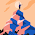# C Program to find Sum of a Series

We are actually going to find the sum of following series:
1 + 2 + 3 + ..... + n
To do so, we need to start a loop from 1 and need to do sum till n. Here is the program:

```#include <stdio.h>

int main()
{
int i, last_num, sum = 0;
printf("Enter the last number of the series: ");
scanf("%d",&last_num);
for(i=1;i<=last_num;i++){
sum = sum + i;
}
printf("The sum of the series is: %d",sum);
return 0;
}```

Now you can do sum of any series with the help of above program.
Share:

#### 1 comments :

1.